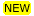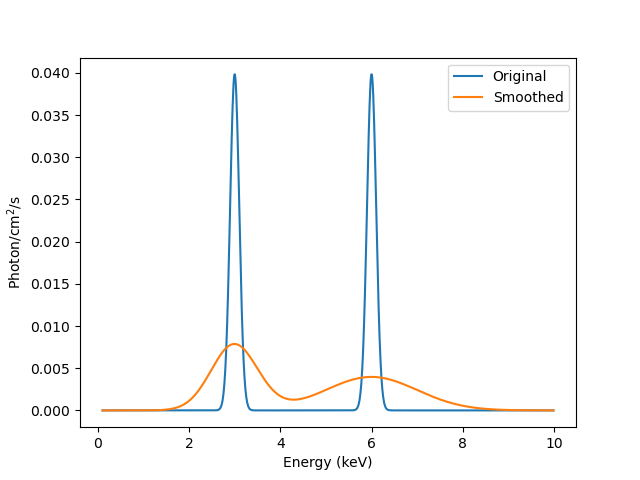# How do I create and use XSPEC convolution models?New in CIAO 4.13 is support for XSPEC convolution models (prior to CIAO 4.13 you could use them via the contributed Python scripts, but this interface has been removed). These models are applied to one or more other model components, which means that their syntax is different to other models (you have to be able to tell the convolution model what model, or models, it should apply to). This is done by function application, as described below.

As an example, the XSPEC xsgsmooth convolution model is used to apply energy-dependent smoothing to a model. For this example we shall create two lines (l1 and l2) which we will then smooth. First the lines:

```sherpa> l1 = create_model_component("xsgaussian", "l1")
sherpa> l2 = create_model_component("xsgaussian", "l2")
sherpa> l1.linee = 3
sherpa> l2.linee = 6
sherpa> mdl = l1 + l2
sherpa> print(mdl)
(xsgaussian.l1 + xsgaussian.l2)
Param        Type          Value          Min          Max      Units
-----        ----          -----          ---          ---      -----
l1.LineE     thawed            3            0        1e+06        keV
l1.Sigma     thawed          0.1            0           10        keV
l1.norm      thawed            1            0        1e+24
l2.LineE     thawed            6            0        1e+06        keV
l2.Sigma     thawed          0.1            0           10        keV
l2.norm      thawed            1            0        1e+24
```

Now the smoothing model:

```sherpa> gsm = create_model_component("xsgsmooth", "gsm")
sherpa> gsm.index = 1
sherpa> print(gsm)
xsgsmooth.gsm
Param        Type          Value          Min          Max      Units
-----        ----          -----          ---          ---      -----
gsm.SigAt6keV thawed            1            0           10        keV
gsm.Index    frozen            1           -1            1
```

We then "apply" the convolution to the model to be convolved using function application, which creates a new model:

```sherpa> convolved = gsm(mdl)
sherpa> print(convolved)
xsgsmooth.gsm((xsgaussian.l1 + xsgaussian.l2))
Param        Type          Value          Min          Max      Units
-----        ----          -----          ---          ---      -----
gsm.SigAt6keV thawed            1            0           10        keV
gsm.Index    frozen            1           -1            1
l1.LineE     thawed            3            0        1e+06        keV
l1.Sigma     thawed          0.1            0           10        keV
l1.norm      thawed            1            0        1e+24
l2.LineE     thawed            6            0        1e+06        keV
l2.Sigma     thawed          0.1            0           10        keV
l2.norm      thawed            1            0        1e+24
```

We can then use this new model in a set_source call, or evaluate it directly to create Figure 1:

```sherpa> egrid = np.arange(0.1, 10, 0.01)
sherpa> elo = egrid[:-1]
sherpa> ehi = egrid[1:]
sherpa> emid = (elo + ehi) / 2
sherpa> yorig = mdl(elo, ehi)
sherpa> yconv = convolved(elo, ehi)
sherpa> plt.plot(emid, yorig, label='Original')
sherpa> plt.plot(emid, yconv, label='Smoothed')
sherpa> plt.legend()
sherpa> plt.xlabel('Energy (keV)')
sherpa> plt.ylabel('Photon/cm\$^2\$/s')
```

### Energy-dependent smoothing### Energy-dependent smoothing

The blue line - labelled Original - shows the output of mdl(elo, ehi), and the orange line the convolved output (that is, convolved(elo, ehi)). The energy-dependent smoothing (since the index parameter of the xsgsmooth component was set to 1) is visible, with the smoothing increasing with energy.

We can check that the convolution has not significantly altered the source signal (there are always edge effects and numerical issues to be aware of):

```sherpa> print(f"Original total:  {yorig.sum()}")
Original total:  2.0
sherpa> print(f"Convolved total: {yconv.sum()}")
Convolved total: 1.9999591588322894
```Note

Convolved models can be combined with other models, so it would be valid to say

```sherpa> set_source(xsphabs.gal * (xsgmooth.gsm(xsgaussian.line) + xsapec.src))
```